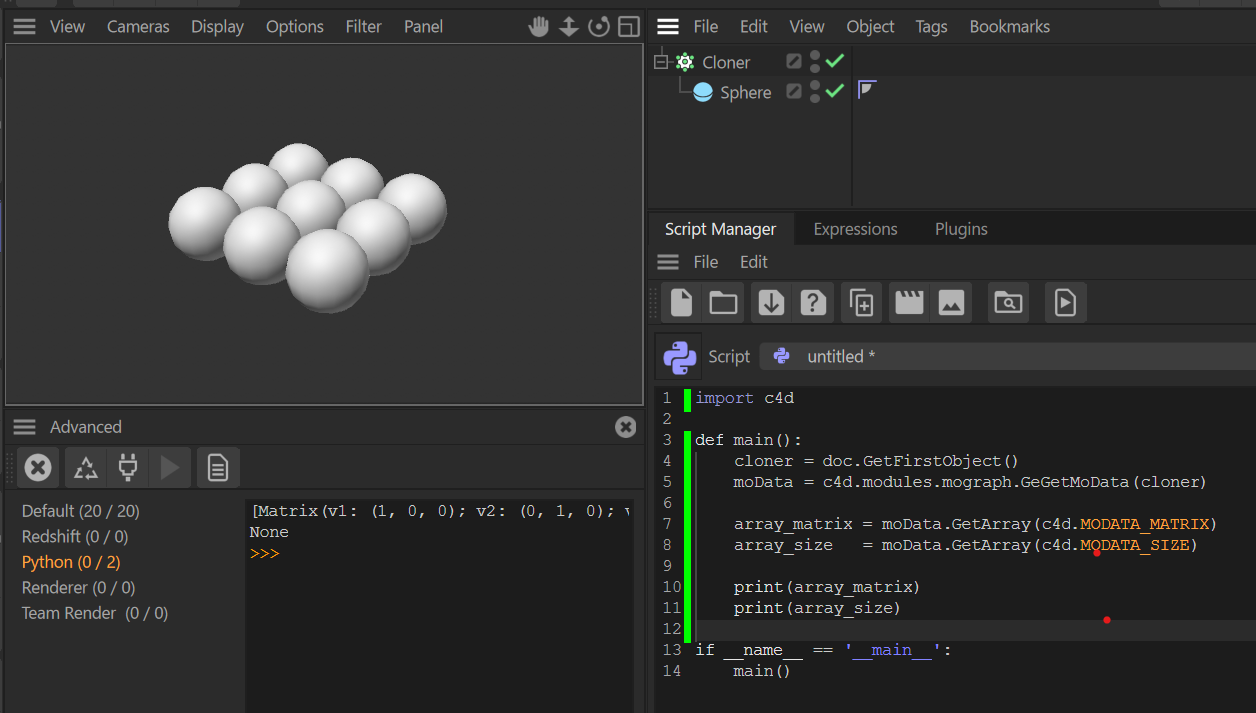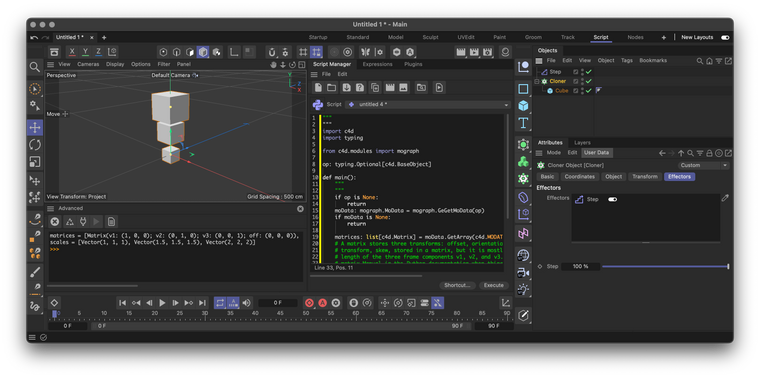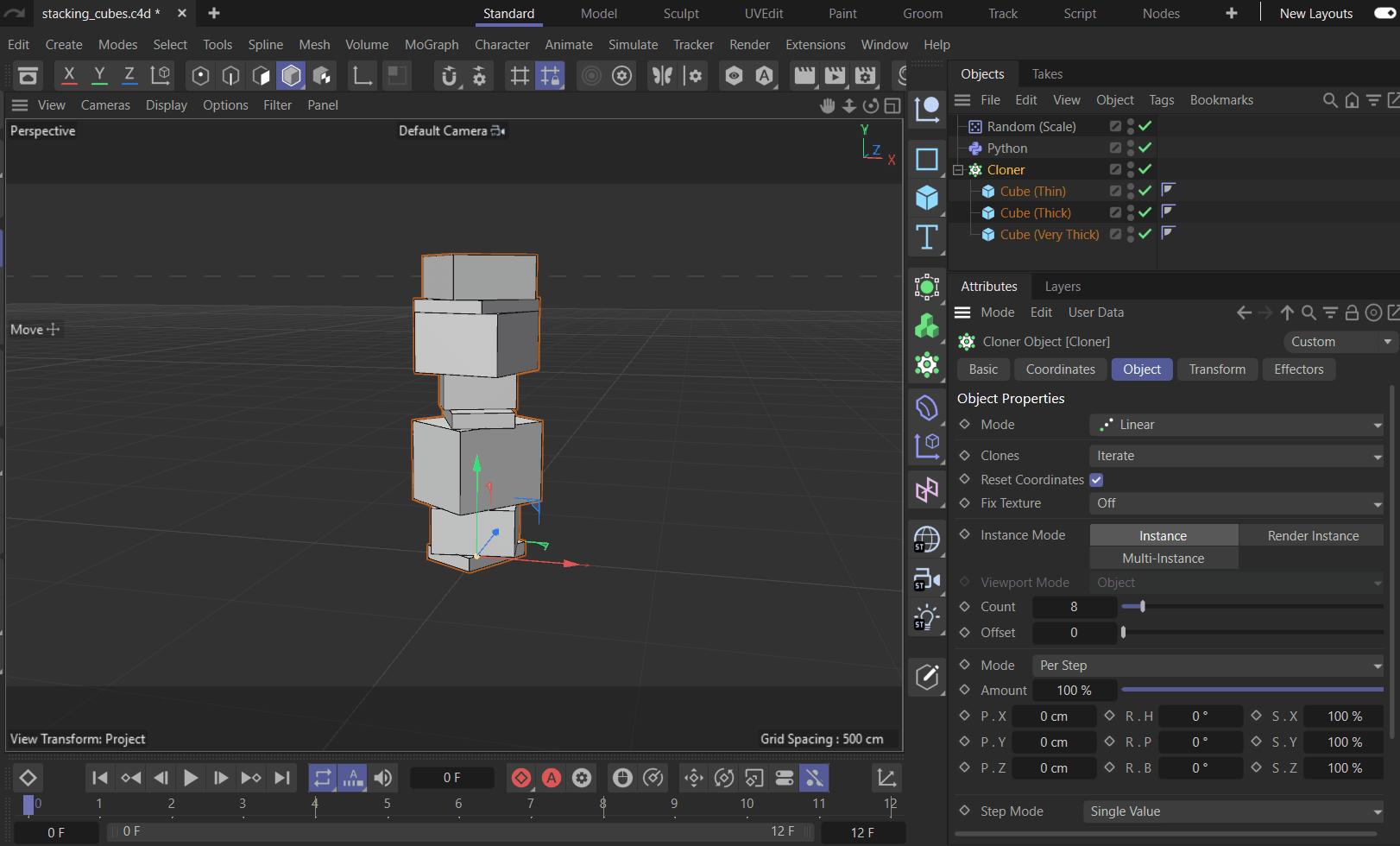# Get MODATA_SIZE of Mograph Particles to Build Stacks of Geometry

Hi,

I tried to get the sizes of clones with `MoData.GetArray(c4d.MODATA_SIZE)`, but it returns None where the other IDs (e.g. `c4d.MODATA_MATRIX`) works fine.

Is this an issue or am I doing something wrong? Any advice would be appreciated.Hello @kng_ito,

Thank you for reaching out to us. `MOGRAPH_SIZE` is only used by the MoGraph Matrix object. If you want the scale of particles, it is encoded in the matrices. The size of particles, i.e., the absolute size values of the geometry attached to a particle, is not accessible, as MoGraph is just a particle system which does not store that information. Find an example at the end of this post.

PS: Please post code. While we appreciate screenshots, it is not fun to copy code from a screenshot.

Cheers,
Ferdinand

Edit: I forogot that there is also Matrix.GetScale, so that you do not have to access the components yourself. But it should not make too much of a difference, unless you have 100,000 or more MoGraph particles, then you should use the builtin method to optimize performance.

The result:The code:

``````"""
"""
import c4d
import typing

from c4d.modules import mograph

op: typing.Optional[c4d.BaseObject]

def main():
"""
"""
if op is None:
return
moData: mograph.MoData = mograph.GeGetMoData(op)
if moData is None:
return

matrices: list[c4d.Matrix] = moData.GetArray(c4d.MODATA_MATRIX)
# A matrix stores three transforms: offset, orientation, and scale (there is actually a fourth
# transform, skew, stored in a matrix, but it is mostly irrelevant). The scale is encoded in the
# length of the three frame components v1, v2, and v3. I would recommend having a look at the
# matrix Manual in the Python documentation when things seem unclear.
#
# So, if one wants a list of nice scale vectors, one could grab them like this.
scales: list[c4d.Vector] = [
c4d.Vector(m.v1.GetLength(), m.v2.GetLength(), m.v3.GetLength()) for m in matrices]

print (f"{matrices = }")
print (f"{scales = }")

if __name__=='__main__':
main()
``````

MAXON SDK Specialist
developers.maxon.net

Hi @ferdinand,

I am not sure I should continue in this thread or should open a new one, but I would like to ask about the way to access the actual size of clones.

I am trying to create a system of stacking cubes whose scale animates, and I need to get the size of each clone to do so. Currently, the size of each clone is obtained by caching the cloner, but this causes processing delays and animation problems.

The scene file:
StackingCubes_ProcessingDelayIssue.c4d

The Python Effector code:

``````import c4d

def main():
moData = c4d.modules.mograph.GeGetMoData(op)
marr = moData.GetArray(c4d.MODATA_MATRIX)

cloner = moData.GetGenerator()
cached = cloner.GetCache()
cubes = cached.GetChildren()

pos_y = 0
for i, cube in enumerate(cubes):
pos_y += height / 2
marr[i].off = c4d.Vector(0, pos_y, 0)
pos_y += height / 2

moData.SetArray(c4d.MODATA_MATRIX, marr, False)
return True
``````

Is there any way to get the size of clones without processing delay?
Any advice or examples similar to this topic would be appreciated.
Thank you.

Hey @kng_ito,

Thank you for your reply. I think it could go both ways, your follow-up question could be a new thread, or a remain here. Because it is Monday, and I am still a bit weekend-lazy, I opted for staying here and adjusting the thread title a bit.

About your code and what you want to do: There are already commercial plugin solutions which solve that stacking thingy. If you want more features, it can get a bit involved, but nothing too hard IMHO. I however opted here for a very naive solution in my answer, which does what your question demanded: stacking cubes generators along the y-axis with no orientation randomization allowed except for the stacking-axis (y).

Your code had the right idea, and you were almost there, but you did two things incorrectly:

1. The children of a cloner do not correspond 1:1 to the particle geometry, and you must retrieve for each particle its indexed clone geometry so that you can do your offset computations.
2. Unless I am a bit Monday-brain-dead, the math in your loop does not quite pan out for me too. You want to offset each particle by half its height plus the offset, and then increment the offset by the full particle height (at least when the bounding box origin is equal to geometry object origin assumption holds true).

As hinted at, there are also other problems with this, most notably that your code always assumes the origin of the particle geometry to lie on the origin of its bounding box (which is true for most generators, but usually not true for hand-made geometry), and the inability of this effector to compute the height of an arbitrarily rotated geometry. I did not tackle both problems in my answer, as they would have bloated the example and are also technically out of scope of support, at least the last problem. If you want to implement that and struggle with it, I would have to ask you to open new threads for these questions.

Cheers,
Ferdinand

The file: stacking_cubes.c4d

The result:The code:

``````"""Simple example for an effector that 'stacks' clones by their particle geometry size.

This can get quite complicated when supposed to be done with arbitrary particle transforms where
particles are rotated in odd angles. This is just a naïve variant stacking along the y-axis,
assuming all particles to have an orientation of (0, y, 0), i.e., only allowing for rotations around
the stacking axis.

Your code had basically the correct idea, multiply the particle scale by the actual geometry size,
but had some problems in the details.
"""
import c4d
import math
import typing

from c4d.modules import mograph

op: c4d.BaseObject # The Python effector object.

# The geometry associated with a Mograph particle is stored as a floating point value, to retrieve
# the index of the cloner child associated with that floating point number, this formula must be
# used.
CloneOffset2Index: typing.Callable[[float, int], int] = lambda offset, count: (
int(math.floor(c4d.utils.ClampValue(offset * count, 0, count - 1)))
)

def main() -> None:
"""Implements the 'stacking' effector.
"""
# Get Mograph data, the particle matrices, and the particle clone offsets.
moData: mograph.MoData = mograph.GeGetMoData(op)
cloneMatrices: list[c4d.Matrix] = moData.GetArray(c4d.MODATA_MATRIX)
cloneOffsets: list[float] = moData.GetArray(c4d.MODATA_CLONE)
if len(cloneMatrices) != len(cloneOffsets):
raise IndexError("Clone matrix and index arrays are not of equal length.")

# Get the cloner and its children, the to be cloned geometry.
cloner: c4d.BaseObject = moData.GetGenerator()
cloneGeometries: list[c4d.BaseObject] = cloner.GetChildren()
cloneGeometriesCount: int = len(cloneGeometries)

# Convert cloneOffsets to integer values. cloneIndices[n] will now contain the child index
# for cloneMatrices[n], i.e., cloneGeometries[cloneIndices[n]] is the geometry for the particle
# n with the matrix cloneMatrices[n].
cloneIndices: list[int] = [
CloneOffset2Index(offset, cloneGeometriesCount) for offset in cloneOffsets]

# Apart from not properly dealing with the clone geometry, your code had also some math problems
# in the loop.

# The offset vector for the clones, so that we can collect the 'stacking' information.
offset: c4d.Vector = c4d.Vector()

# Iterate over all clones as clone matrix, clone geometry index pairs.
for cloneMatrix, cloneIndex in zip(cloneMatrices, cloneIndices):
# Get the y-scale of the particle and half the size of the geometry bounding box along
# the y-axis and construct a vector with it, representing half the height of the actual
# particle geometry.
particleScaleY: float = cloneMatrix.GetScale().y
localOffset: c4d.Vector = c4d.Vector(0, particleScaleY * geometryRadiusY, 0)

# Offset the clone by half its height on top of the current offset, so that it sits on top
# of whatever was below it. And after that, increment the offset by twice that value, as we
# want to respect the full particle in the offset.
cloneMatrix.off = offset + localOffset
offset += localOffset * 2

# What we did here in the last step is a very lack-lustre implementation, as it assumes the
# origin of a particle geometry to always be located on the arithmetic mean of its bounding
# box minima/maxima, i.e, that it always sits "in the middle". This assumption holds true
# for things like primitive generators, but will quickly fail on "real world" geometry which
# can place its origin almost anywhere. To make this effector more robust, you will have to
# evaluate the delta between the origin of the geometry in world coordinates and the bounding
# box origin (BaseObject.GetMp()) in world coordinates and respect that in your calculations.
# I left this here out because I wanted the example to be A. simple, and because B. we cannot
# provide full solutions.

# What has also not been respected in this script, is the orientation of particles, you could
# for example want to stack cubes which are "balancing" on one of their edges, this here only
# works for staccking geometry on the top and bottom faces of their bounding boxes.

moData.SetArray(c4d.MODATA_MATRIX, cloneMatrices, False)
return True
``````

MAXON SDK Specialist
developers.maxon.net

Hi @ferdinand,

I expected there to be a way to know which particle referred to which original geometry, but I didn't know how, so thanks. I should have looked more closely at the documentation. Moreover, I didn't think it could handle blended cloners.

Anyway, thank you very much. Problem solved.